# Weirdness , Trying to guess price action with a moving average and 4 deltas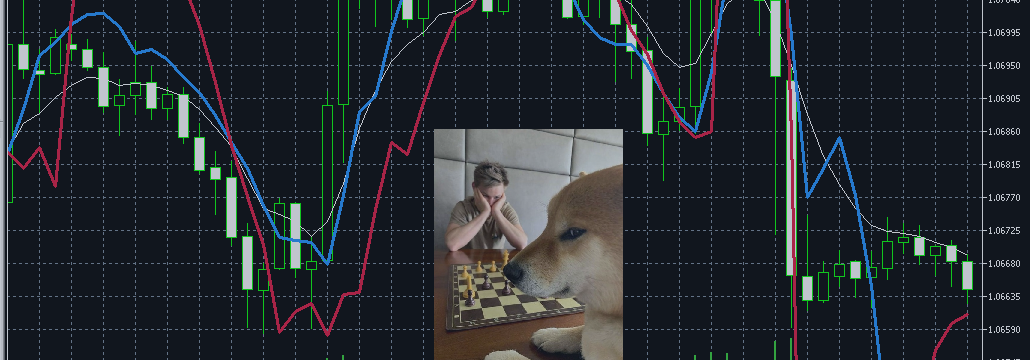That is a quick write up , here is the code :

```#property version   "1.00"
#property indicator_chart_window
#property indicator_buffers 10
#property indicator_plots   1
input int SpinePeriod=4;
input ENUM_APPLIED_PRICE SpinePrice=PRICE_MEDIAN;
input int SlowsPeriod=200;
input int FastsPeriod=7;
input string noteA="STYLING";
input color SpineColor=clrWhite;
input ENUM_LINE_STYLE SpineStyle=STYLE_SOLID;
input int SpineWidth=1;

double Spine[],spinePeriod[];
double DeltaHighToSpine[],DeltaLowToSpine[];
double SlowHighToSpineAvg[],SlowLowToSpineAvg[],SlowPeriods[];
double FastHighToSpineAvg[],FastLowToSpineAvg[],FastPeriods[];
bool RatesSeries=false;
double FullSpine=0.0,FullSlow=0.0,FullFast=0.0;

int OnInit()
{

int co=0;
RatesSeries=false;
SetIndexBuffer(co,Spine,INDICATOR_DATA);
PlotIndexSetInteger(co,PLOT_DRAW_TYPE,DRAW_LINE);
PlotIndexSetInteger(co,PLOT_LINE_COLOR,SpineColor);
PlotIndexSetInteger(co,PLOT_LINE_STYLE,SpineStyle);
PlotIndexSetInteger(co,PLOT_LINE_WIDTH,SpineWidth);
co++;
SetIndexBuffer(co,spinePeriod,INDICATOR_DATA);
PlotIndexSetInteger(co,PLOT_DRAW_TYPE,DRAW_NONE);
co++;
SetIndexBuffer(co,DeltaHighToSpine,INDICATOR_DATA);
PlotIndexSetInteger(co,PLOT_DRAW_TYPE,DRAW_NONE);
co++;
SetIndexBuffer(co,DeltaLowToSpine,INDICATOR_DATA);
PlotIndexSetInteger(co,PLOT_DRAW_TYPE,DRAW_NONE);
co++;
SetIndexBuffer(co,SlowHighToSpineAvg,INDICATOR_DATA);
PlotIndexSetInteger(co,PLOT_DRAW_TYPE,DRAW_NONE);
co++;
SetIndexBuffer(co,SlowLowToSpineAvg,INDICATOR_DATA);
PlotIndexSetInteger(co,PLOT_DRAW_TYPE,DRAW_NONE);
co++;
SetIndexBuffer(co,SlowPeriods,INDICATOR_DATA);
PlotIndexSetInteger(co,PLOT_DRAW_TYPE,DRAW_NONE);
co++;
SetIndexBuffer(co,FastHighToSpineAvg,INDICATOR_DATA);
PlotIndexSetInteger(co,PLOT_DRAW_TYPE,DRAW_NONE);
co++;
SetIndexBuffer(co,FastLowToSpineAvg,INDICATOR_DATA);
PlotIndexSetInteger(co,PLOT_DRAW_TYPE,DRAW_NONE);
co++;
SetIndexBuffer(co,FastPeriods,INDICATOR_DATA);
PlotIndexSetInteger(co,PLOT_DRAW_TYPE,DRAW_NONE);
FullSpine=SpinePeriod;
FullFast=FastsPeriod;
FullSlow=SlowsPeriod;

return(INIT_SUCCEEDED);
}

int OnCalculate(const int rates_total,
const int prev_calculated,
const datetime &time[],
const double &open[],
const double &high[],
const double &low[],
const double &close[],
const long &tick_volume[],
const long &volume[],
{
if(SymbolIsSynchronized(_Symbol)){

int from=prev_calculated+1,to=rates_total;
if(prev_calculated==0){

if(ArrayIsSeries(open)){RatesSeries=true;}
ArrayFill(Spine,0,ArraySize(Spine),0.0);
ArrayFill(spinePeriod,0,ArraySize(spinePeriod),0.0);
ArrayFill(DeltaHighToSpine,0,ArraySize(DeltaHighToSpine),0.0);
ArrayFill(DeltaLowToSpine,0,ArraySize(DeltaLowToSpine),0.0);
ArrayFill(SlowHighToSpineAvg,0,ArraySize(SlowHighToSpineAvg),0.0);
ArrayFill(SlowLowToSpineAvg,0,ArraySize(SlowLowToSpineAvg),0.0);
ArrayFill(SlowPeriods,0,ArraySize(SlowPeriods),0.0);
ArrayFill(FastHighToSpineAvg,0,ArraySize(FastHighToSpineAvg),0.0);
ArrayFill(FastLowToSpineAvg,0,ArraySize(FastLowToSpineAvg),0.0);
ArrayFill(FastPeriods,0,ArraySize(FastPeriods),0.0);
}

for(int i=from;i<rates_total;i++){
int j=i;
if(!RatesSeries){j=rates_total-i-1;}

spinePeriod[i]=spinePeriod[i-1];
Spine[i]=Spine[i-1];

if(spinePeriod[i]==FullSpine){
DeltaHighToSpine[i]=MathAbs(high[j]-Spine[i]);
DeltaLowToSpine[i]=MathAbs(low[j]-Spine[i]);

SlowHighToSpineAvg[i]=SlowHighToSpineAvg[i-1];
SlowLowToSpineAvg[i]=SlowLowToSpineAvg[i-1];
SlowPeriods[i]=SlowPeriods[i-1];
FastHighToSpineAvg[i]=FastHighToSpineAvg[i-1];
FastLowToSpineAvg[i]=FastLowToSpineAvg[i-1];
FastPeriods[i]=FastPeriods[i-1];

SlowPeriods[i]=SlowPeriods[i-1];

FastPeriods[i]=FastPeriods[i-1];

}

}

return(rates_total);
}return(0);
}

double get_prices(const double open,const double high,const double low,const double close,ENUM_APPLIED_PRICE applied){
double price=0.0;
if(applied==PRICE_CLOSE){price=close;}
else if(applied==PRICE_HIGH){price=high;}
else if(applied==PRICE_LOW){price=low;}
else if(applied==PRICE_OPEN){price=open;}
else if(applied==PRICE_MEDIAN){price=NormalizeDouble((high+low)/2.00,_Digits);}
else if(applied==PRICE_TYPICAL){price=NormalizeDouble((high+low+close)/3.00,_Digits);}
else if(applied==PRICE_WEIGHTED){price=NormalizeDouble((high+low+close+close)/4.00,_Digits);}
return(price);
}
void add_to_rolling_average(double data,double &_total,int max,double &avg){
_total+=1.0;
int t=(int)MathRound(_total);

if(t>max){

avg=avg-(avg/((double)max))+(data/((double)max));
_total=max;
}

else if(t==max){
avg+=data;
avg/=((double)max);
}

else{
avg+=data;
}
}```

We have the spine average , rolling .

When we have enough spine periods we start collecting spine deltas.

We also rolling average them

Now here is the weird attempt.

We want to create an index which when added on the spine it comes as close as possible to the actual high prices.

We will call this highGuess . Same for the lows , lowGuess.

So let’s establish more rules :

• we start “optimizing” when we have full periods on everything

We are not interested in what happened each yesterday with yesterdays spine and yesterdays guesses , like analysts are . Nonono , we want

the yesterdays spine to project todays high and lows , and if it fails we won’t hide it! So rule 2

Now let’s introduce more weirdness . We have fasts and slows right ?

If we have a plot that is a composite of the slow and fast and a toggle variable that adjusts the weights of how much fast and how much slow the

plot is we can get a fluctuating average , won’t be accurate , but , won’t be slow either (in crunch time).

So let’s call that X . If X is the weight , the % , the amount of Fasts in the new plot then 1.00 – X is the % , the amount of Slows in the Plot.

That is very handy as this is one unknown !

So , if we have :

1. a completed bar
2. with a previous bar that has all rollings available
3. we know the previous to it spine level
4. we know its high and low
5. we don’t know what X is (or what X should have been)

And this is the weird part , we will use the X of the knowns as the “toggle” for the unknowns and , we will plot the guesses accordingly (including our errors).

After that we could go ahead and also rolling average the X! but one weirdness at a time .

So okay , we want X , how do we get it ?

and for the Lows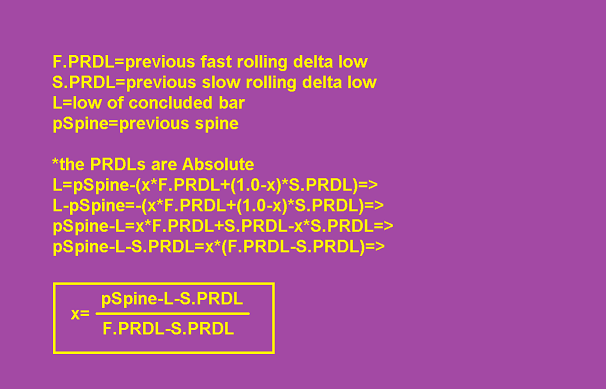If there’s a mistake let me know .

So let’s code it and see what happens.

On first glance its volatile , both guesses .

(white=spine , blue=guess high, red = guess low)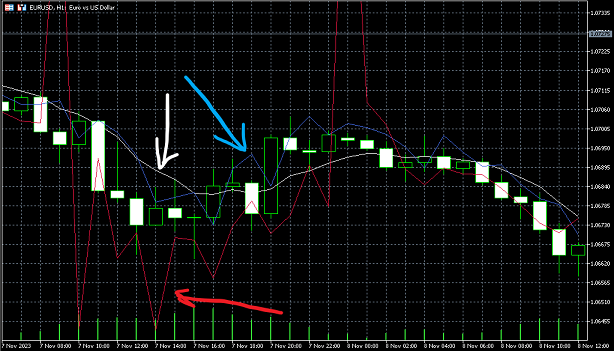So what can we do? We can try adding a rolling average to the x coefficients as well.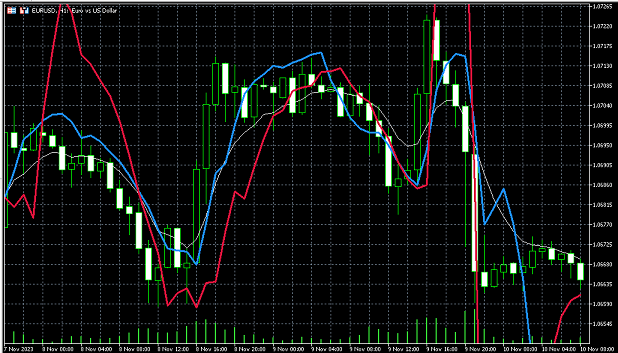hmm not much better xD . Anyway that was fun.

attached is the source code.

–EDITS—–

But hang on , we are getting high projections that are below the spine .

Why ? because the projection is based on the previous spine and we will only know the current spine once the bar concludes .

There is no point in projecting on the spine as it evolves , we want a clear projection at the time of bar opening that says

high is here , low is here . We want to trade it not brag about it being right yesterday .

However , if we place ourselves at the time of a bar’s opening , we do have the open price .! right ?

So , version 2 can be using the open price for both testing and projecting instead of the spine .

Let’s see how it does.

Not much different , but i realized that we have an issue anyway.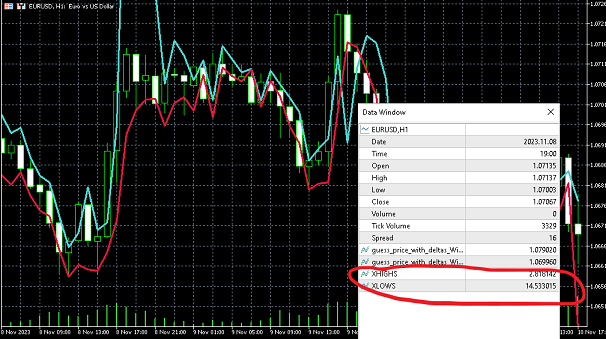the Xs are not bound between 0 and 1 !

i’ll have to come up with something better there.

Anyway attaching the version with opens as well.

Will update when i find a solution